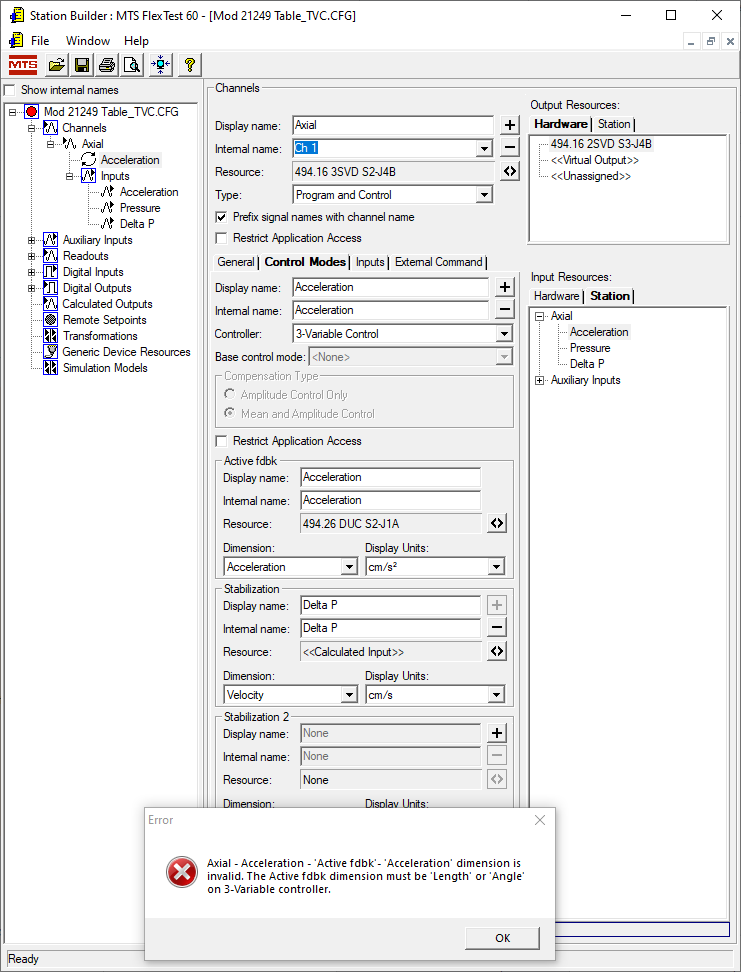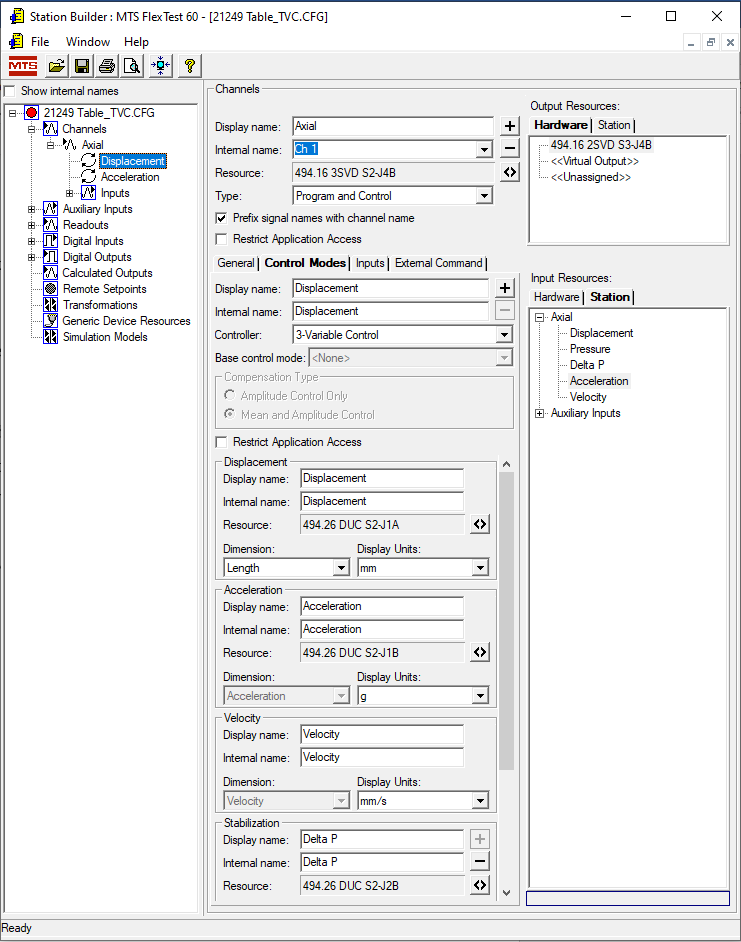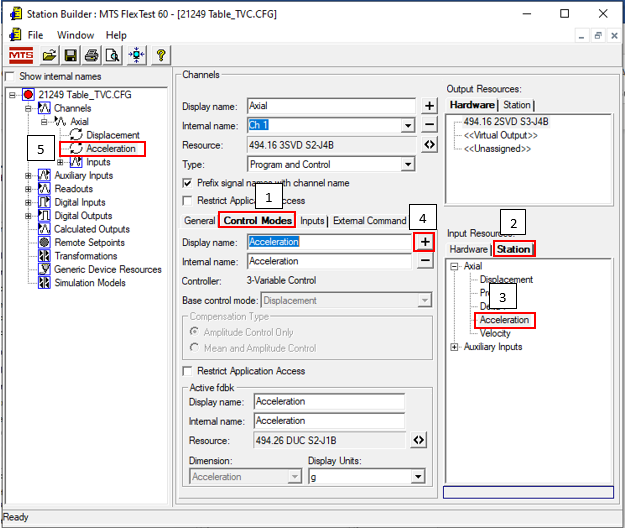# Axial – Acceleration – ‘Active fdbk’ – ‘Acceleration’ dimension is invalid. The Active fdbk dimensioin must be ‘Length’ or ‘Angle’ on 3- Variable controller.

PROBLEM

Trying to create Three Variable Control (TVC) with Acceleration as the primary control mode results in the following error window (Figures  1 & 2):

Axial – Acceleration – ‘Active fdbk’ – ‘Acceleration’ dimension is invalid.  The Active fdbk dimensioin must be ‘Length’ or ‘Angle’ on 3- Variable controller.Figure 1 – Error WindowFigure 2 – 3-Variable Control (TVC) in Station Setup

You must create the 3-Variable Control (TVC) control mode with Displacement as the primary control mode first (Figure 1).Figure 1 – TVC setup with using Displacement as the primary control mode

1. Click on the Control Modes tab.
2. In the Input Resources select the Station tab and then expand the list for the channel.
3. You should see the signals for Displacement, Acceleration and Velocity.  Select the Acceleration signal.
4.  Click the plus (+) button to add Acceleration as a control mode.
5. You can do the same for Velocity if desired.
6. In the main panel tree view you can re-order the mode by dragging them up or down if desired.Figure 2 – Adding Acceleration as a primary control mode in 3-Variable Control (TVC)

K
Keary is the author of this solution article.

Did you find it helpful? Yes No

Send feedback